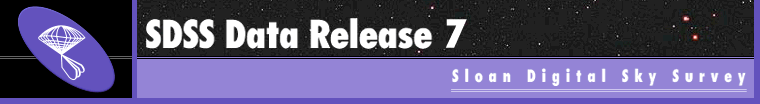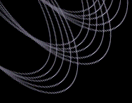Go to sdss.orgDR7 HomeWhere to StartDataDatabase (CAS)Files (DAS)Value-AddedTutorialsOlder ReleasesAbout DR7Sky CoverageInstrumentsData ProcessingAlgorithmsHelpFAQGlossaryKnown IssuesDR PapersHelpdeskSearch# Reading SDSS object lists and checking flags

The catalogs of detected objects in the SDSS imaging data are output in a series of so-called tsObj files. The SDSS sky is divided up into a series of stripes, overlapping great circles on the sky 2.5 degrees wide. Each stripe is observed as a pair of strips, each of which contains six scanlines, images in five bands from each of the columns of CCDs in the imaging camera. The scanlines are 13 arcmin wide, and overlap by roughly one arcminute when the two strips of a stripe are interleaved. Each scanline is divided up, for processing purposes, into frames, consisting of 1361X2048 pixels (10'X13'), and the catalogs of objects from each frame, together with all their attributes, are output in these tsObj files.

The tsObj files are fits binary tables. One can read them using standard FITS IO libraries. Alternatively, one can use routines in IDL, SM, MIDAS, and IRAF to read them (see software for reading fits files and images). What follows is an SM implementation to read a series of tsObj files; it should be easily translatable, e.g., into C code.

Each run of the SDSS imaging camera is given a sequential number; it covers a given strip. The correspondence between run number and strip number is given in the SDSS coverage tables. A tsObj file is named by the run number, a rerun number (i.e., an internal processing number), the camera column number (i.e., which of the six scanlines), and a sequential number indicating the frame in question; thus:
tsObj-000756-1-20-0400.fit (run 756, camera column 1, rerun 20, frame 400).

You can search for objects and find the run, rerun, camcol, and field id of the fields in which they fall using the CAS Imaging Query Service (IQS) Form. If you have a right ascension and declination, you can look up the frame in which it falls with CAS SQL query. For example, this query will list the fields that cover RA=180.5 degrees, declination=12.0 degrees:

```
SELECT DISTINCT f.run, f.rerun, f.camcol, f.field
FROM dbo.fGetNearbyFrameEq(180.0,12.0,23.4,0) AS nf
CROSS APPLY dbo.fCoordsFromEq(180.0,12.0) AS p
JOIN field AS f ON f.fieldID = nf.fieldID
WHERE
((p.mu*f.e_r-f.b_r*p.nu-f.e_r*f.a_r+f.b_r*f.d_r)/(f.c_r*f.e_r-f.b_r*f.f_r))
BETWEEN 0 AND 2048
AND
((p.mu*f.f_r-f.c_r*p.nu-f.a_r*f.f_r+f.c_r*f.d_r)/(f.b_r*f.f_r-f.c_r*f.e_r))
BETWEEN 0 AND 1489
```

The 23.4 in line 3 is the distance from the center of a field to a corner, in arcminutes. The long messy equations calculate the row and column pixel coordinates in the field.

You can also use the DAS coordinate lookup tool to descover the run, rerun, camcol, and field id covering a specific set of coordinates.

The full data model for the tsObj file is given in the tsObj data model. It consists of a single HDU. The header includes information on the mean seeing and sky brightness of the frame in all five bands, and flags indicating whether the fit to the point spread function was good, as well as whether the data were considered to be good, acceptable, bad (usually because of poor seeing) or hole (processing timed out, because of a very bright star or globular cluster). There are roughly 2 kilobytes of attributes per object, as described in detail in the section on the "frames" pipeline of the EDR paper.

We provide example SM code describing an algorithm to pull out a clean sample of stars from the data (see the SM homepage if you don't have SM). Note the extensive use of binary flag bits for all aspects of describing the data, from labelling objects as spectroscopic targets, to giving information on the details of the photometric pipeline processing of the object. Examples are given here on reading the target flags, as well as deriving aperture magnitudes from the data.